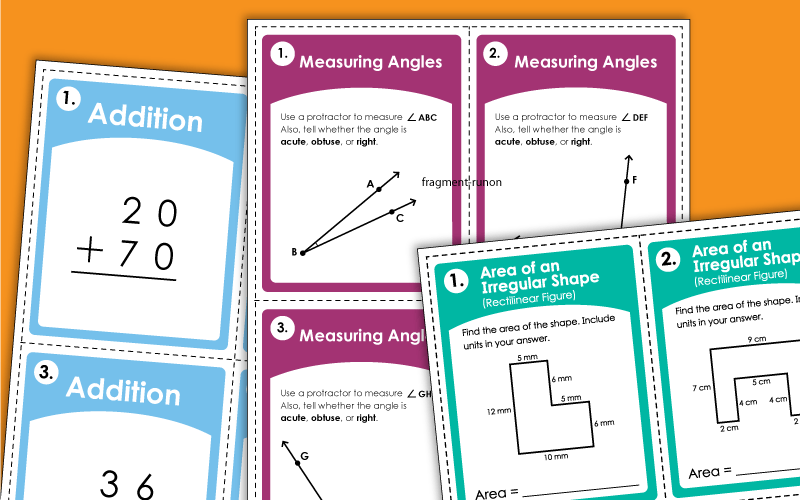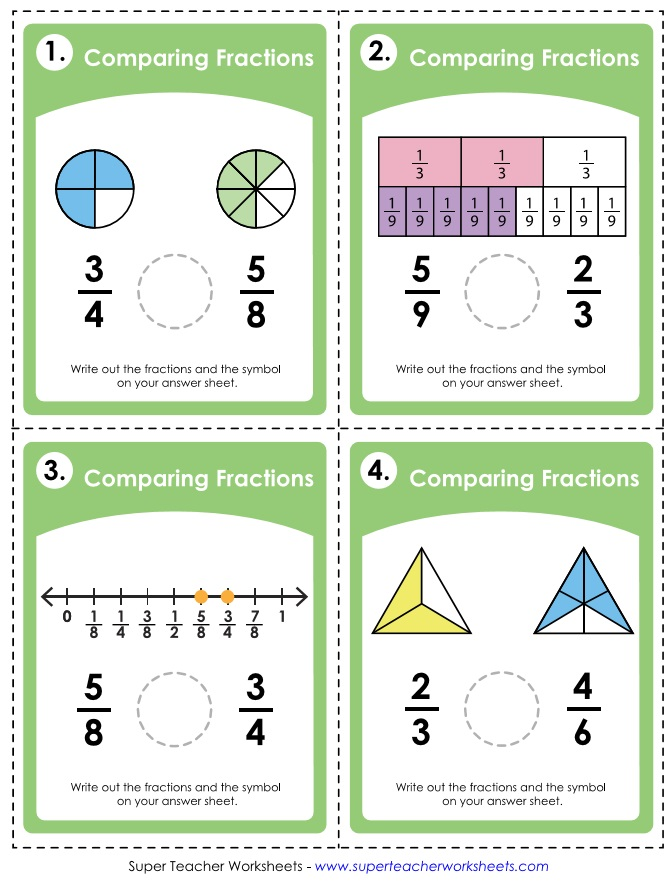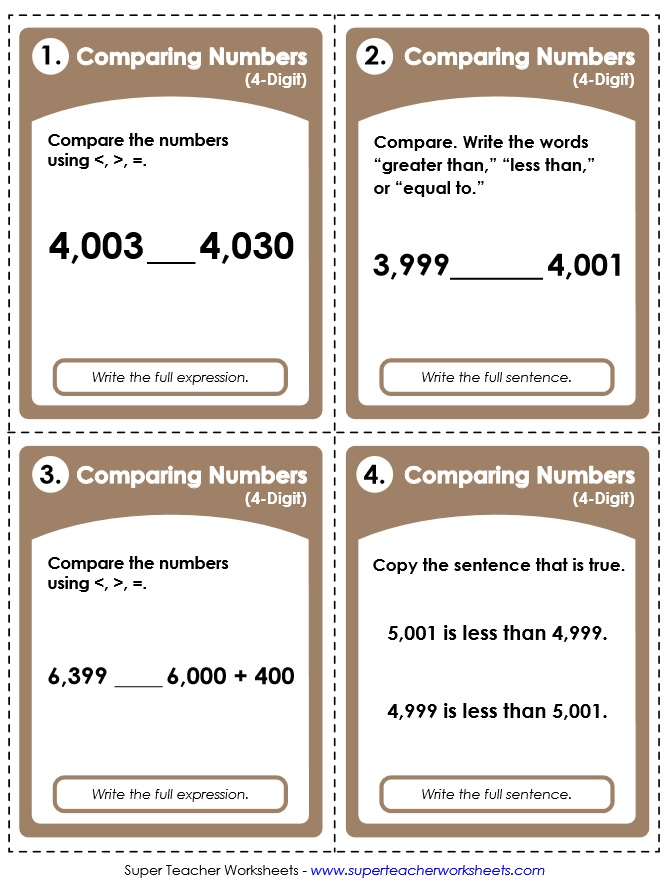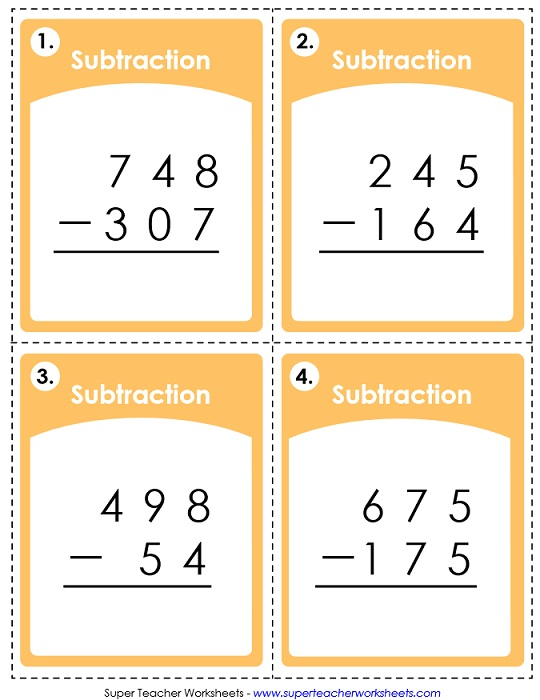# Printable Task Cards

There are countless ways to use the task cards below. You can create classroom learning centers. Post them as daily or weekly challenges. Use them with your whiteboard's document camera. Laminate them and let students write on them with dry-erase markers. The possibilities are endless.This file has 30 task cards for teaching absolute value of integers.
This task card set has 30 addition problems. Students do not regroup (carry) in these problems.
1st and 2nd Grades
These two-digit addition problems will require students to regroup (carry ones).
1st and 2nd Grades
On these cards, students will solve addition problems with addends up to 3 digits.
2nd and 3rd Grades
These task cards have 4-digit addition problems for students to solve.
2nd through 4th Grades
This set of 30 cards can be used to review addition with 5-digit addends. Problems are vertical.
4th and 5th Grades
Look carefully at the base-10 block models and the partial hundreds charts to figure out what is "10 more."
1st and 2nd Grades
Add a multiple of 10 to each 2-digit number. Each card has PV block models or a hundreds chart.
1st and 2nd Grades
Measure each angle with a protractor. Write the measurement of each and tell if it is acute, obtuse, or right.
4th through 6th Grades
Identify whether angles are acute, right, or obtuse using these printable task cards.
4th and 5th Grades
This version of the "counting square units" task cards is slightly harder than the version listed above.
3rd through 5th Grades
Given the radius or diameter, calculate the areas of the circles.
6th through 8th Grades
This set contains "area of a parallelogram" problems.
6th through 8th Grades
Practice finding the area of rectangles by multiplying the length by the width.
3rd through 5th Grades
In this deck you'll find a collection of 30 "area of a trapezoid" problems for students to solve.
6th through 8th Grades
Find the area of the irregular shape (rectilinear figure) by finding the area of each rectangle within the shape and adding those areas together.
3rd through 5th Grades
This set contains 30 task cards. Find the areas of each rectangle within the shape by multiplying decimals. Then add them together to find the area of the irregular shape (rectilinear figure).
3rd through 5th Grades
This math task card set has 30 "greater than / less than / equal to" problems for kids to solve.
1st and 2nd Grades
this task card set will help students practice comparing 3-digit numbers using the symbols for "greater than," less than," and "equal to."
2nd and 3rd Grades
Each of the cards in this set has 4-digit numbers for students to compare.
2nd through 4th Grades
Use these task cards for learning centers or class games. Includes 30 "greater/less/equal" problems with five-digit numbers.
3rd through 5th Grades
These task cards can be used to help students practice using the greater than, less than, and equal to symbols to compare numbers with six digits.
4th and 5th Grades
Write a contraction for the underlined words.
1st through 4th Grades
Identify, name decimals, and write numbers in decimal notation. Includes tenths and hundredths.
3rd through 5th Grades
These cards have 30 basic division problems, with remainders. (example: 8 divided by 38)
3rd through 5th Grades
On these PDF task cards, students will divide numbers with 3-digit dividends. (example: 127 divided by 2)
4th through 6th Grades
With these task cards, students will practice dividing using 2-digit divisors. The problems are written traditionally, horizontally, and as fractions.
3rd through 6th Grades
With the problems on this PDF, you can try your hand at division of mixed numbers.
5th and 6th Grades
Divide decimals by 10, 100, and 1,000 with these printable task cards.
4th and 5th Grades
Learn about exponents with this set. Write each repeated multiplication fact as an exponent. Then compare exponents. Then write exponents in standard form.
5th through 8th Grades
Students will determine which basic fractions are shown by the illustrations on the cards.
2nd through 4th Grades
Compare pairs of fractions. Use the illustration models to help.
3rd through 5th Grades
Compare pairs of fractions using the mathematical symbols <, >, and =.
3rd through 5th Grades
This is a set of 30 task cards on simplifying (reducing) fractions.
3rd through 5th Grades
Add fractions with unlike denominators and write the sum on the answer page.
4th through 6th Grades
Solve fraction subtraction problems with unlike denominators. Simplify your answers.
4th through 6th Grades
Find the least common denominator (LCD) for each pair of fractions.
4th and 5th Grades
Calculate the fraction of a set. For example: What is 5/6 of 48? This set has 30 cards, which includes 15 word problems.
4th through 6th Grades
Switch improper fractions to mixed numbers, and mixed numbers to improper fractions.
3rd through 5th Grades
Choose the correct work (you're or your) to complete the sentences shown.
2nd through 4th Grades
Solve these mixed number addition problems with unlike denominators. Don't forget to simplify your answers!
4th through 6th Grades
A set of 30 task cards to practice subtracting mixed numbers with unlike denominators.
4th through 6th Grades
On these task cards, students will find the values of the coins shown. Each card shows only a few, easy-to-count coins. Example: A dime and a penny = \$0.11
1st through 3rd Grades
Add up the values of the coins to find the total. (example: 3 quarters + 2 dimes + 3 pennies = \$0.98)
1st through 3rd Grades
Your students will use these cards alone, in small groups, or as a class and count the bills and coins to find the amount. Example: 2 one dollar bills + 2 quarters + 2 pennies = \$2.28.
2nd through 4th Grades
Round money amounts up to the next dollar. Example: To buy a sweater that costs \$8.19, you would need \$9.00.
1st and 2nd Grades
For these activities, students must find the missing factor for each basic multiplication fact.
2nd through 4th Grades
Students write the basic multiplication fact that corresponds with the arrays shown. (Basic facts 0s through 9s.)
2nd through 4th Grades
Multiply double-digit numbers by single-digit numbers on these 30 task cards.
3rd and 4th Grades
Multiply three-digit by 1-digit numbers on these printable task cards.
3rd and 4th Grades
With these printable task cards students can find products of pairs of 2-digit numbers.
4th through 6th Grades
With these cards, students can try to multiply pairs of three-digit numbers.
4th through 6th Grades
Practice multiplying decimals to the tenths and hundredths place by single digit numbers with the help of these task cards.
4th through 6th Grades
With these printable task cards, students will practice multiplying two digit numbers by decimals to the tenths and hundredths.
4th through 6th Grades
On these cards, students will practice multiplying pairs of fractions.
5th and 6th Grades
With these task cards, your students can review multiplication of mixed numbers.
5th and 6th Grades
These problems cards have money amounts as the first factor, and a single-digit number for the second factor. (example: \$4.56x3)
3rd and 4th Grades
Read the sentences on the cards. Each one has exactly one noun in it. Identify the nouns.
1st through 3rd Grades
Here are discussion cards that can be used for discussion of any short story, novel, or chapter book.
3rd through 6th Grades
Here are 30 cards with 2-digit numbers. Students round each number to the nearest ten.
(example: Round 37 to the nearest ten.)
2nd and 3rd Grades
Round each 2 or 3-digit number to the nearest ten. (example: Round 148 to the nearest ten.)
3rd and 4th Grades
Round each number to the nearest hundred. (example: Round 329 to the nearest hundred.)
3rd and 4th Grades
Round each 3 or 4-digit number number to the nearest hundred. (example: Round 2,310 to the nearest hundred.)
3rd through 5th Grades
These have a mixture of 2 3, and 4-digit numbers. Round to the nearest ten or hundred, as per the instructions.
3rd through 5th Grades
Round each of these numbers to the nearest tens, hundreds, or thousands place.
3rd through 5th Grades
Round each number to the nearest tenth using these printable task cards
4th through 6th Grades
Round the each number to the nearest whole number, tenth, or hundredth.
4th through 6th Grades
With these cards, students will practice subtracting two-digit numbers. These problems do not require students to regroup of borrow.
1st and 2nd Grades
Subtract pairs of 2-digit numbers. Most of these problems require students to regroup (borrow).
2nd and 3rd Grades
Subtract pairs of three-digit numbers on these student task cards. Most problems require students to borrow/regroup/rename.
2nd through 4th Grades
With these 30 task cards, students can practice subtracting 4-digit numbers.
3rd through 5th Grades
Here you'll find 4-digit subtraction problems. All require students to borrow across the number zero.
3rd through 5th Grades
Subtract 10 from 2-digit numbers using the place value block models and the partial hundreds charts.
(example: 35-10)
1st and 2nd Grades
Subtract multiples of ten from 2-digit numbers using a hundreds chart or base-10 blocks.
(examples: 87-40=47 and 92-50=42)
1st and 2nd Grades
This set has 12 cards with clocks on them. The clocks show "top of the hour" times. (examples: 3:00, 7:00, 12:00)
Kindergarten and 1st Grade
This one has 24 different clocks. Each one shows a time in half-hour intervals. (examples: 5:30, 12:00, 1:30, 9:00)
Kindergarten and 1st Grade
These clocks have times with 5 minute intervals. (example: 8:50, 10:25, 1:05) There are thirty clocks in this set.
1st through 3rd Grades
On these cards, you'll see clocks with one-minute intervals.(examples: 1:34, 12:57, 9:02) Students must read the clock and tell what time is shown.
3rd through 5th Grades
Determine how many cubes are in each shape to estimate the volume.
3rd through 5th Grades
Calculate the volumes of the given cylinders. Includes 15 cards with picture models and 15 phrase problems.
7th and 8th Grades
Multiply length times width times height to find the volumes of the rectangular prisms.
4th through 6th Grades
This is an advanced-level worksheets for calculating volumes of rectangular prisms. Unlike the basic level, each shape has one or more decimal measurements.
5th and 6th Grades

Scoot! Games

This page has Scoot! games. This is a whole-class card game for practicing math concepts.

"I have / Who has" Games

"I have... Who has..." is a whole-class card game in which students answer math facts quickly without breaking the chain.

Classroom Scavenger Hunts

For this game, students search the classroom for hidden facts or math problems to solve.

## Images of Our PrintablesMy Account
Site Information